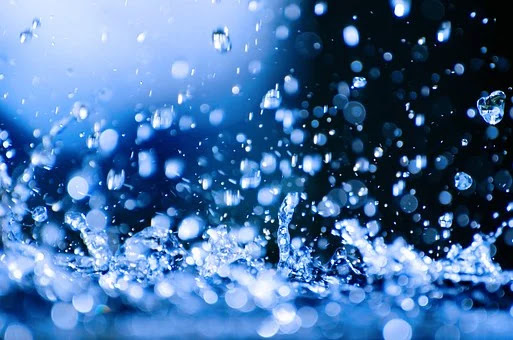# Objective Questions | Properties of Fluid | MCQs | Fluid Mechanics | By Ashutosh Nautiyal

1. Which of the following is considered as an ideal fluid? a. Mercury b. Kerosene c. Benzene d. Water Answer. d (Water) 2. Printer's ink is an

## Objective Questions (Properties of Fluid)Objective Questions (Properties of Fluid)

a. Mercury
b. Kerosene
c. Benzene
d. Water

## 2. Printer's ink is an

a. Newtonian fluid.
b. Non-Newtonian fluid.
c. Thixotropic fluid.
d. Ideal Plastic fluid.

## 3. Poise is the unit of

a. Kinematic Viscosity.
b. Mass Density.
c. Dynamic Viscosity.
d. None of the above.

## 4. Raindrops are spherical due to

a. Surface tension.
b. Viscosity.
c. Air resistance.
d. Atmospheric pressure.

## 5. Stoke is the unit of

a. Kinematic viscosity.
b. Dynamic viscosity.
c. Surface tension.
d. None of the above.

a. Air
b. Hydrogen
c. Mercury
d. Water

## 7. Which of the following quantities is dimensionless?

a. Specific Gravity
b. Specific Volume
c. Specific Mass
d. Specific Weight

## 8. Which of the following is incorrect?

a. Density has the units of mass per unit volume.
b. Specific Weight has units of weight per unit volume.
c. Shear stress has the units of force per unit area.
d. Specific Gravity has the units of mass per unit volume.

Answer. d (Specific Gravity has the units of mass per unit volume.)

## 9. The phenomenon of Capillarity is due to

a. Cohesion.
d. None of the above.

a. kg/m2s.
b. kgs/m2.
c. m/kgs.
d. m2/s.

## 11. Falling drops of water become spherical due to

a. Cohesion.
c. Viscosity.
d. Surface Tension.

a. N/m2.
b. m/N.
c. N/m.
d. m2/N.

## 13. The Specific Gravity of water is

a. 9.81
b. 1
c. 0
d. None of the above

a. 100
b. 1
c. 10
d. 1000

a. 1
b. 1000
c. 10
d. 10000

## 16. Mercury is considered the best liquid to be used in pressure measuring device because it has

a. low vapour pressure and high density.
b. high vapour pressure and high density.
c. low surface tension.
d. high density and low viscosity.

Answer. a (low vapour pressure and high density)

## 17. N/m is the unit of

a. Viscosity.
b. Capillarity.
c. Surface Tension.
d. Compressibility.

a. 4 °C
b. 0 °C
c. 273 K.
d. 300 K.

a. ρ/μ
b. μ/ρ
c. μρ
d. μ/g

## 20. If the Cohesion between molecules of a liquid is greater than the Adhesion between liquid and glass tube, the free level of liquid in the dipped glass tube will be

a. lower than the general level of the liquid.
b. higher than the general level of the liquid.
c. same as the general level of the liquid.
d. None of the above.

Answer. a (lower than the general level of the liquid.)

## 21. Real fluids are

a. viscous.
b. compressible.
c. possess surface tension.
d. All of the above.

Answer. d (All of the above.)

## 22. Bulk Modulus of Elasticity is the ratio of the

a. compressive stress to compressive strain.
b. compressive stress to volumetric strain.
c. tensile strain to tensile strain.
d. None of the above.

Answer. b (compressive stress to volumetric strain.)

## 23. The Specific Weight of water in the SI unit is

a. 1000 N/m3.
b. 9810 N/m3.
c. 9.81 N/m3.
d. 1000 kg/m3.

## 24. The increase in temperature

a. increases the viscosity of a liquid.
b. decreases the viscosity of a gas.
c. decreases the viscosity of a liquid.
d. None of the above.

Answer. c (decreases the viscosity of a gas.)

## 25. Mercury does not wet the glass tube. It is due to the property of liquid known as

b. Viscosity.
c. Cohesion.
d. Surface Tension.

## 26. The Mass Density of water in the SI unit is

a. 1000 kg/m3.
b. 9810 kg/m3.
c. 1 kg/m3.
d. 9.81 kg/m3.

## 27. The ratio of the Specific Weight of the liquid to the Specific Weight of a standard liquid at a standard temperature is known as

a. Weight Density.
b. Specific Gravity.
c. Specific Volume.
d. Viscosity.

## 28. The study of liquids, in motion, when pressure forces are considered, is called

a. Hydrostatic.
b. Hydrokinematics.
c. Hydrodynamics.
d. None of the above.

a. Nsm.
b. Ns/m2.
c. Ns/m.
d. N/sm.

## 30.  The force of attraction between like molecules is called

a. Capillarity.
c. Surface Tension.
d. Cohesion.

*Self Typed
*Source- Books, Internet, Self-Analysis

Getting Info...